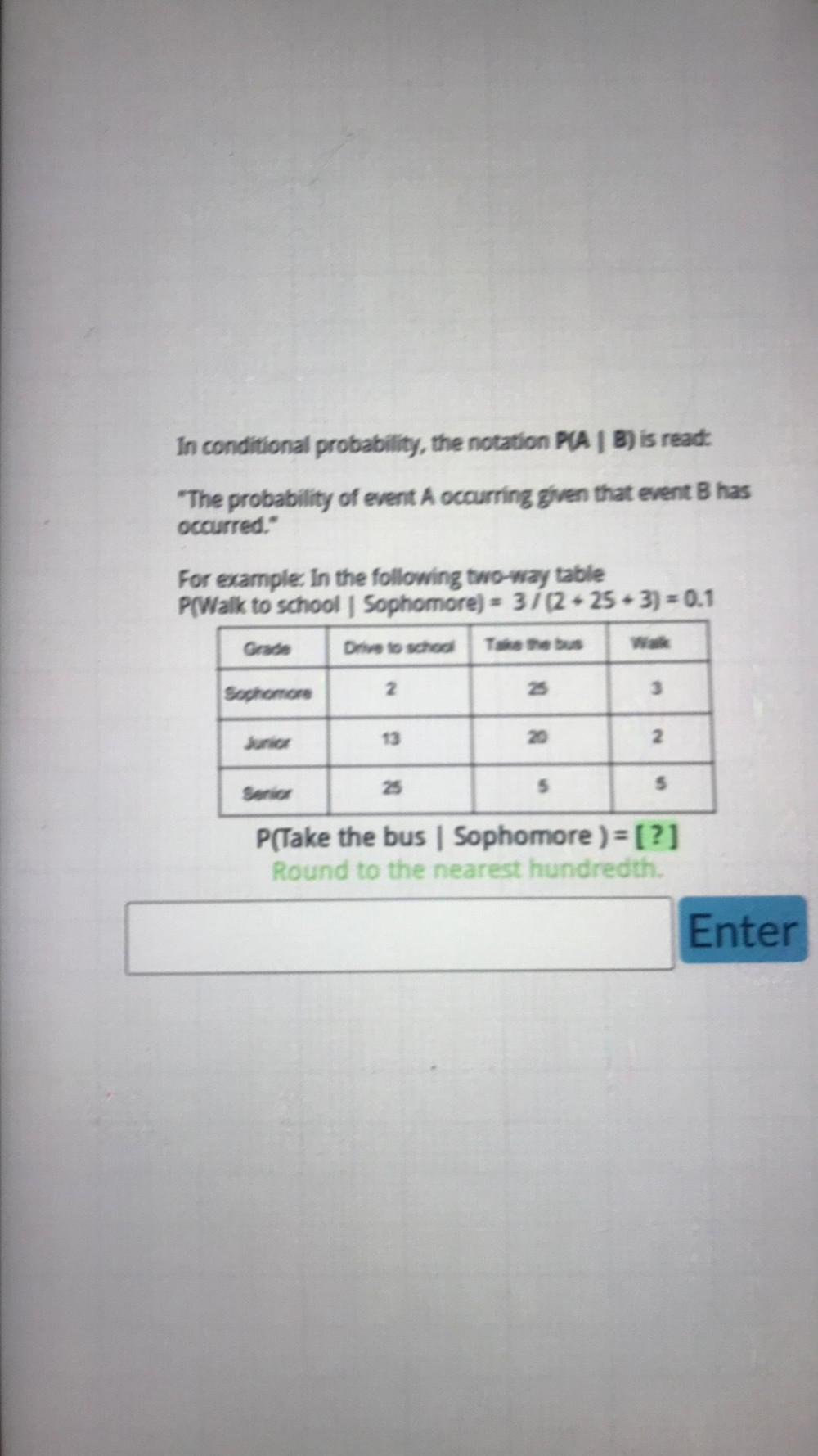Question:

# In conditional probability, the notation P(A/B) is read: "The probability of event A occurring given that event B has occurred." For example: the following two-way table P(Walk to school Sophomore) =In conditional probability, the notation P(A/B) is read: "The probability of event A occurring given that event B has occurred." For example: the following two-way table P(Walk to school Sophomore) = 3/(2-25 + 3) = 0.1 Grade Drive to school Take the bus Walk Sophomore 2 25 3 Junior 13 20 2 Senior 25 5 5 P(Take the bus | Sophomore ) = [?]